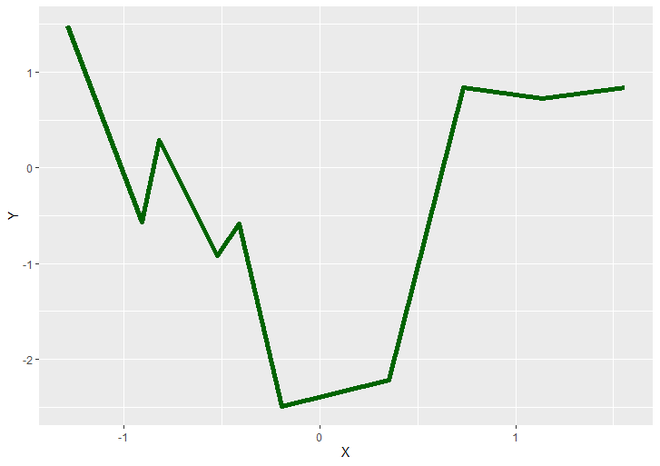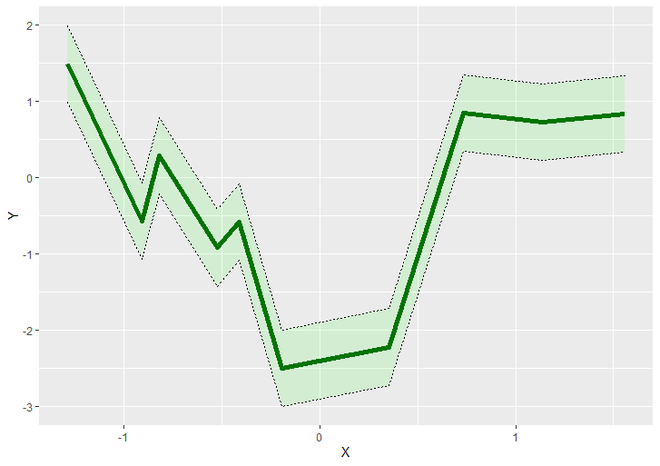Related Articles

# Shading confidence intervals manually with ggplot2 in R

• Last Updated : 30 Jun, 2021

In this article, we will see how to generate Shading confidence intervals manually with ggplot2 in R Programming language.

Let us first draw a regular curve and then add confidence intervals to it.

Example:

## R

 `# Load Packages``library``(``"ggplot2"``) `` ` `# Create a DataFrame for Plotting``DF <- ``data.frame``(X = ``rnorm``(10),                                   ``                 ``Y = ``rnorm``(10))`` ` `# Plot the ggplot2 plot``ggplot``(DF, ``aes``(X, Y)) +                                     ``  ``geom_line``(color = ``"dark green"``, size = 2)`

Output:LineGraph using ggplot2

To add shading confidence intervals, geom_ribbon() function is used. Which displays a Y interval defined by ymin and ymax. It has aesthetic mappings of ymin and ymax. Other than that it also has some more parameters which are not necessary.

Syntax : geom_ribbon(mapping, color, fill, linetype, alpha, …)

Parameters :

• mapping : aesthetic created by aes() to define ymin and ymax.
• color : Specifies the color of border of the Shading interval.
• fill : Specifies the color of Shading Confidence Interval.
• linetype : Specifies the linetype of the border of Confidence Interval.
• alpha : Specifies the Opacity of the Shading Interval.
• … : geom_ribbon also has other parameters such as data, stat, position, show.legend, etc. You can use them as per your requirements but in general case they are not useful as much.

Return : Y interval with the specified range.

Example:

## R

 `# Load Packages``library``(``"ggplot2"``) `` ` `# Create DataFrame for Plotting``DF <- ``data.frame``(X = ``rnorm``(10),                                   ``                 ``Y = ``rnorm``(10))`` ` `# ggplot2 LineGraph with Shading Confidence Interval``ggplot``(DF, ``aes``(X, Y)) +                                     ``  ``geom_line``(color = ``"dark green"``, size = 2) +``  ``geom_ribbon``(``aes``(ymin=Y+0.5, ymax=Y-0.5), alpha=0.1, fill = ``"green"``, ``              ``color = ``"black"``, linetype = ``"dotted"``)`

Output: